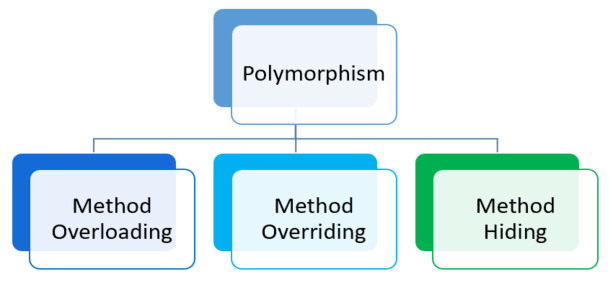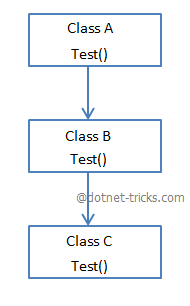# Understanding virtual, override and new keyword in C#

22 Aug 2022
Intermediate
83.4K Views

Polymorphism is one one of the main aspect of OOPS Principles which include method overriding and method overloading. Virtual and Override keyword are used for method overriding and new keyword is used for method hiding. In this article, In this article, I am going to explain each keyword in details with the help of C# code.## Simple Class Inheritance

Consider the below class hierarchy with classes A, B, and C. A is the super/base class, B is derived from class A and C is derived from class B.If a method Test() is declared in the base class A and classes B or C has no methods as shown below.

```using System;
namespace Polymorphism
{
class A
{
public void Test() { Console.WriteLine("A::Test()"); }
}

class B : A { }

class C : B { }

class Program
{
static void Main(string[] args)
{
A a = new A();
a.Test(); // output --> "A::Test()"

B b = new B();
b.Test(); // output --> "A::Test()"

C c = new C();
c.Test(); // output --> "A::Test()"

}
}
}
```

Suppose you have Test() method in all the classes A, B, C as shown below:

```using System;
namespace Polymorphism
{
class A
{
public void Test() { Console.WriteLine("A::Test()"); }
}

class B : A
{
public void Test() { Console.WriteLine("B::Test()"); }
}

class C : B
{
public void Test() { Console.WriteLine("C::Test()"); }
}

class Program
{
static void Main(string[] args)
{

A a = new A();
B b = new B();
C c = new C();

a.Test(); // output --> "A::Test()"
b.Test(); // output --> "B::Test()"
c.Test(); // output --> "C::Test()"

a = new B();
a.Test(); // output --> "A::Test()"

b = new C();
b.Test(); // output --> "B::Test()"

}
}
}
```

When you will run the above program, it will run successfully and give the O/P. But this program will show the two warnings as shown below:

1. 'Polymorphism.B.Test()' hides inherited member 'Polymorphism.A.Test()'. Use the new keyword if hiding was intended.

2. 'Polymorphism.C.Test()' hides inherited member 'Polymorphism.B.Test()'. Use the new keyword if hiding was intended.

## Method Hiding (new keyword)

As you have seen in the above example the compiler generates the warnings since C# also supports method hiding. For hiding the base class method from the derived class simply declare the derived class method with a new keyword. Hence above code can be re-written as :

```using System;
namespace Polymorphism
{
class A
{
public void Test() { Console.WriteLine("A::Test()"); }
}

class B : A
{
public new void Test() { Console.WriteLine("B::Test()"); }
}

class C : B
{
public new void Test() { Console.WriteLine("C::Test()"); }
}

class Program
{
static void Main(string[] args)
{

A a = new A();
B b = new B();
C c = new C();

a.Test(); // output --> "A::Test()"
b.Test(); // output --> "B::Test()"
c.Test(); // output --> "C::Test()"

a = new B();
a.Test(); // output --> "A::Test()"

b = new C();
b.Test(); // output --> "B::Test()"

}
}
}
```

Moreover, if you are expecting the fourth and fifth output should be "B::Foo()" and "C::Foo()" since the objects a and b are referenced by the object of B and C respectively then you have to re-write the above code for `Method Overriding`.

## Method Overriding (virtual and override keyword)

In C#, for overriding the base class method in a derived class, you have to declare a base class method as `virtual` and derived class method as`override` shown below:

```using System;
namespace Polymorphism
{
class A
{
public virtual void Test() { Console.WriteLine("A::Test()"); }
}

class B : A
{
public override void Test() { Console.WriteLine("B::Test()"); }
}

class C : B
{
public override void Test() { Console.WriteLine("C::Test()"); }
}

class Program
{
static void Main(string[] args)
{

A a = new A();
B b = new B();
C c = new C();
a.Test(); // output --> "A::Test()"
b.Test(); // output --> "B::Test()"
c.Test(); // output --> "C::Test()"

a = new B();
a.Test(); // output --> "B::Test()"

b = new C();
b.Test(); // output --> "C::Test()"

}
}
}
```

## Mixing Method Overriding and Method Hiding

You can also mix the method hiding and method overriding by using virtual and new keyword since the method of a derived class can be virtual and new at the same time. This is required when you want to further override the derived class method into the next level as I am overriding Class B, Test() method in Class C as shown below:

```using System;
namespace Polymorphism
{
class A
{
public void Test() { Console.WriteLine("A::Test()"); }
}

class B : A
{
public new virtual void Test() { Console.WriteLine("B::Test()"); }
}

class C : B
{
public override void Test() { Console.WriteLine("C::Test()"); }
}

class Program
{
static void Main(string[] args)
{

A a = new A();
B b = new B();
C c = new C();

a.Test(); // output --> "A::Test()"
b.Test(); // output --> "B::Test()"
c.Test(); // output --> "C::Test()"

a = new B();
a.Test(); // output --> "A::Test()"

b = new C();
b.Test(); // output --> "C::Test()"

}
}
}
```

#### Note

1. The virtual keyword is used to modify a method, property, indexer, or event declared in the base class and allow it to be overridden in the derived class.

2. The override keyword is used to extend or modify a virtual/abstract method, property, indexer, or event of base class into a derived class.

3. The new keyword is used to hide a method, property, indexer, or event of base class into derived class.

Read More Articles Related to CSharp